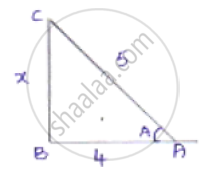Share

# In the Following, Trigonometric Ratios Are Given. Find the Values of the Other Trigonometric Ratios. Cos a = 4/5 - CBSE Class 10 - Mathematics

#### Question

In the following, trigonometric ratios are given. Find the values of the other trigonometric ratios.

cos A = 4/5

#### Solution

We know that cos theta = "adjacent side"/"hypotenuse"

Let us consider a right-angled ΔABCLet opposite side BC = x.

By applying Pythagoras theorem, we get

𝐴𝐶2 = 𝐴𝐵2 + 𝐵𝐶2

25 = x + 16

x = 25 - 16 = 9

x = sqrt9 = 3

We know that cosA = 4/5

sin A = "opposite side"/"hypotenuse" = 3/5

tan A = "opposite side"/"adjacent side"  = 3/4

cosec A = 1/(sin A) = (1/3)/5 = 5/3

sec A = 1/(cos A) = (1/4)/5 = 5/4

cot A = 1/(tan A) =(1/3)/4 = 4/3

Is there an error in this question or solution?

#### APPEARS IN

Solution In the Following, Trigonometric Ratios Are Given. Find the Values of the Other Trigonometric Ratios. Cos a = 4/5 Concept: Trigonometric Ratios.
S